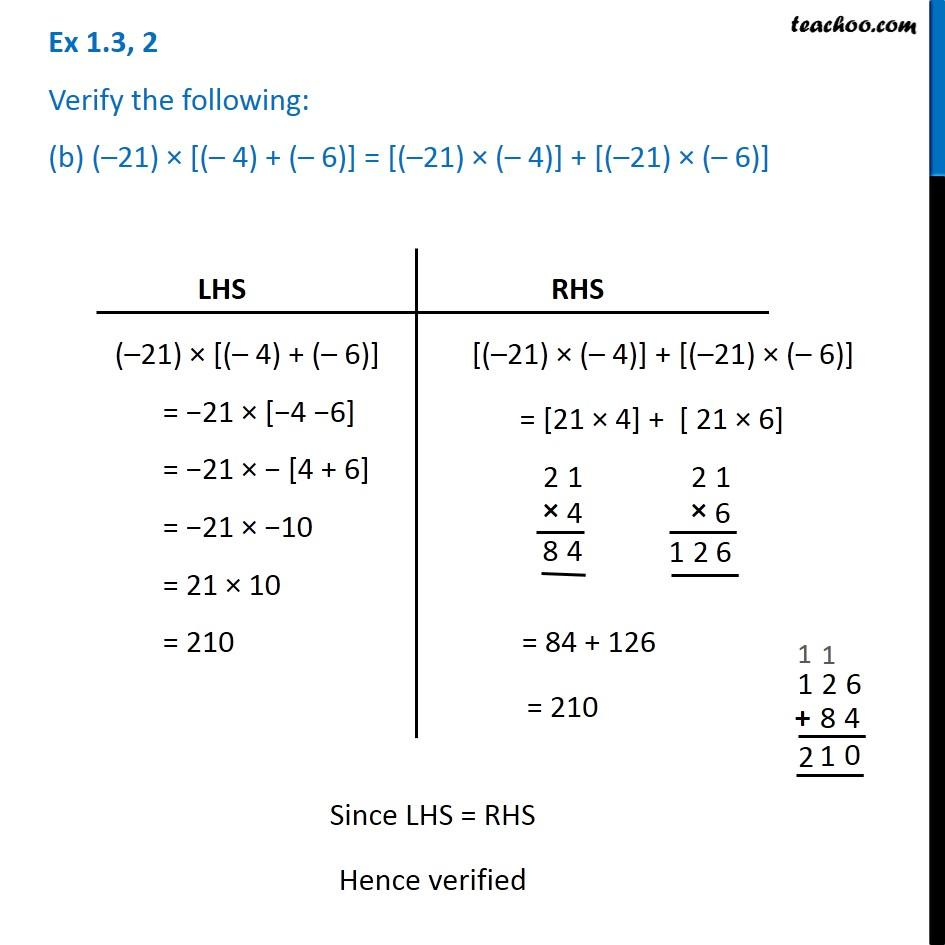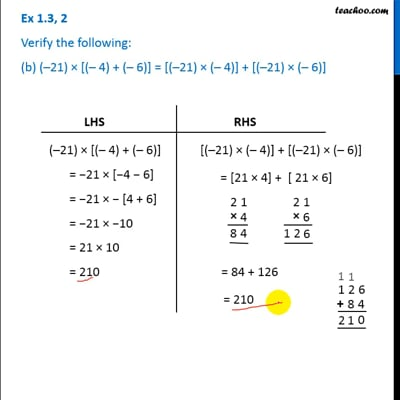Ex 1.3

Chapter 1 Class 7 Integers
Serial order wiseThis video is only available for Teachoo black users

### Transcript

Ex 1.3, 2 Verify the following: (b) (–21) × [(– 4) + (– 6)] = [(–21) × (– 4)] + [(–21) × (– 6)] LHS (–21) × [(– 4) + (– 6)] = −21 × [−4 −6] = −21 × − [4 + 6] = −21 × −10 = 21 × 10 = 210 RHS [(–21) × (– 4)] + [(–21) × (– 6)] = [21 × 4] + [ 21 × 6] = 84 + 126 = 210 Since LHS = RHS Hence verified